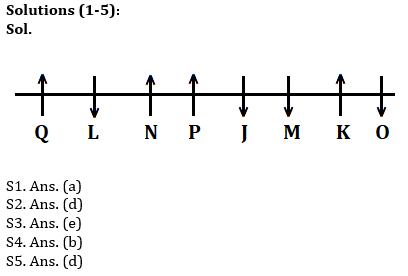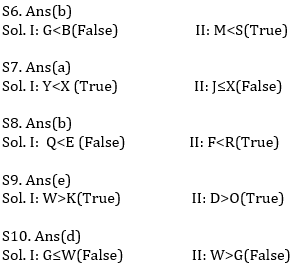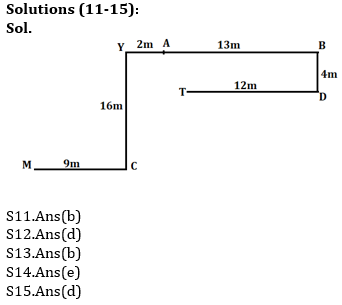Latest Banking jobs   »   NIACL AO 2021 Result Out, Mains Exam Date For 300 AO Recruitment   »   Reasoning Ability Quiz For IBPS Clerk/NIACL...

# Reasoning Ability Quiz For IBPS Clerk/NIACL AO Prelims 2021- 21st September

Directions (1-5): Study the information carefully and answer the questions given below.
Eight persons J, K, L, M, N, O, P and Q are sitting in a linear row some of them facing towards the north and some of them facing towards south but not necessary in the same order. Not more than two consecutive persons face same direction.
N sits second to the right of J. Q sits second to the left of N. Two persons sit between Q and P. More than three persons sit between L and O. The persons sit on extreme ends face opposite direction to each other. M sits second to the right of P. N is not immediate neighbor of M, who sits third from one of the extreme ends of row. O is not immediate neighbor of N and M. O face south direction. J sits immediate right of M. L face opposite direction to Q.

Q1. Who among the following person sits second to right of O?
(a)M
(b)P
(c)J
(d)K
(e)None of these

Q2. How many person sits between N and K?
(a) One
(b) Two
(c) Four
(d) Three
(e)None of these

Q3. Who among the following person is immediate neighbor of P and J?
(a) L
(b) M
(c) K
(d) Q
(e) None of these

Q4. How many persons sits on the right of M?
(a) One
(b) More than Four
(c) Three
(d) Four
(e) None

Q5. Four of the following five are alike in certain way based from a group, find the one that does not belong to that group?
(a) Q
(b) N
(c) K
(d) J
(e) P

Directions (6-10): In these questions, relationship between different elements is show in the statements. The statements are followed by conclusions. Study the conclusions based on the given statements and select the appropriate answer:
(a) If only conclusion I follows.
(b) If only conclusion II follows.
(c) If either conclusion I or II follows
(d) If neither conclusion I nor II follows.
(e) If both conclusions I and II follow.

Q6. Statements: W>G=H≤B>S=D>N≥M
Conclusion I: G<B      II: M<S

Q7. Statements: Y<T=X≥B>N=D>H≥J
Conclusion I: Y<X      II: J≤X

Q8. Statements: Q≥C>R=P>F<E>M≤O
Conclusion I: Q<E      II: F<R

Q9. Statements: O<Q=V=D>W>G=Y≥J>K
Conclusion I: W>K     II: D>O

Q10. Statements: W>P =Z>J <G≥I>O≥T=Q >S
Conclusion I: G≤W   II: W>G

Directions (11-15): Study the given information and answer the following question.
Point A is 13m west of point B. Point D is 4m south of point B. Point C is east of 9m of point M. Point T is 12m west of point D. Point Y is 16m north of point C. Point Y is 2m west of point A.Q12. Point Y is in which direction with respect to D?
(a) North-East
(b) East
(c) West
(d) North-West
(e) None of these

Q13. If Point K is 3m east of point C then what is the shortest distance between Point T and Point K?
(a) 18m
(b) 12m
(c) 9m
(d) 7m
(e) None of these

Q14. What is the direction of C with respect of T?
(a) North-west
(b) South
(c) North-east
(d) West
(e) None of these

Q15. In which direction of Point A with respect to Point T?
(a) North
(b) North-east
(c) East
(d) North-west
(e) None of these

SolutionsClick Here to Register for Bank Exams 2021 Preparation Material#### Congratulations!Download Hindu Review of October 2021: Free PDF